Select Page

# Checksum in Error Detection

A Checksum is a redundancy bits which are appended with actual data for error detection.

At sender side the checksum is generated and at receiver side checksum is validated. Block diagram of Checksum is given below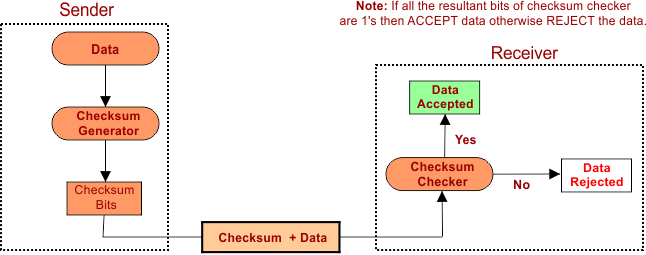## 1. Checksum at Sender Side

At sender side checksum is generated through following steps

Step 01: Break the original data into “K” number of blocks with “N” bits in each block.

Step 02: Sum all the “K” data blocks

Step 03: Add the carry bit if it exists.

Step 04: Find the 1st Complement

The result after 4th step, Checksum is ready to append with data.

## 2. Checksum at Receiver Side

For validation of data at sender side follow the following steps

Step 01: Sum all the “K” data blocks and Checksum

Step 02: Add carry bits if any

Step 03: If the result is all 1’s, ACCEPT data otherwise REJECT the data.

### Explain with Example

Consider the data unit 10011001111000100010010010000100. Now apply the above steps

### 1. Checksum Generator at Sender Side

Step No 1: Break the original data into “K” number of equal size blocks.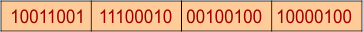Step No 2: Sum all “K” Blocks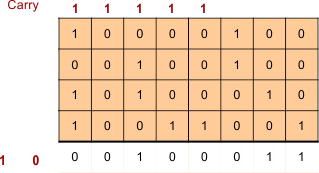Step No 3: Add carry if any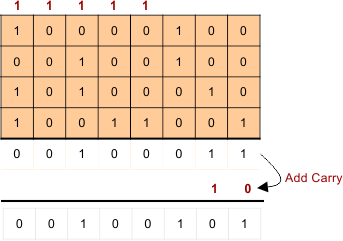Step No 4: Find the 1st Complement = Checksum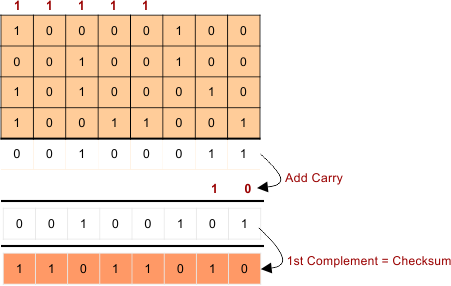### 2. Checksum Validation at Receiver Side

Step 01: Sum all the “K” data blocks and Checksum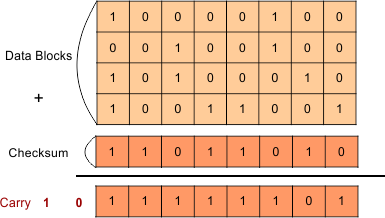Step 02: Add carry if any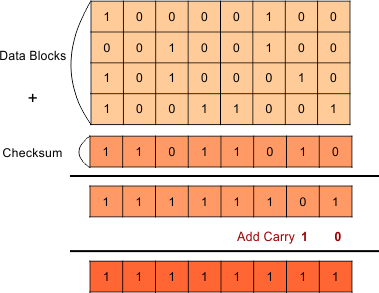Step 03: If all the resultant bits of step 2 are 1’s, ACCEPT data otherwise REJECT the data.

As we see the result of step 2 is all 1’s so data is accepted.

## Performance of Checksum

• Checksum can detect even or odd bits changing.
• If one or more bits of a data block are changed and corresponding bit or bits of opposite value in a second block are also changed, the sum of those columns will not change. So, error will not detect.

Help Other’s By Sharing…